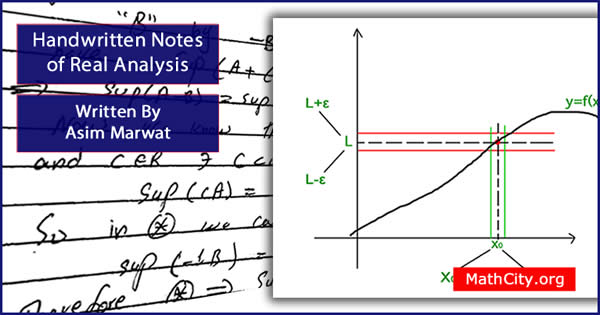# Handwritten Notes of Real Analysis by Asim MarwatReal analysis is a branch of mathematics that analyses how real numbers, sequences and series, and real functions behave. It focuses on real numbers and frequently extends the real line by including positive and negative infinity. Real analysis investigates a number of the properties of real-valued sequences and functions, including convergence, limits, continuity, smoothness, differentiability, and integrability.

The field of mathematics known as “real analysis” was created to describe the study of real numbers and functions as well as to explore fundamental ideas like limits and continuity. These concepts form the foundation of calculus and its applications. Real analysis is becoming a crucial tool in a wide range of applications.

These are basic notes useful in MSc or BS mathematics for real analysis. We appreciate Asim Marwat for making these notes available and for making the effort to publish them on MathCity.org.

 Name Handwritten Notes of Real Analysis Asim Marwat PDF (see Software section for PDF Reader) Different chapter have different size
• Chapter 01: Real Numbers
• Bounded above set, least upper bound (lub), bounded below set, greatest lower bound (glb), Archimedean property, density theorem, Bolzano Weierstrass theorem.
• Chapter 02: Limit of the Function
• Sequence, bounded sequence, Sandwhich theorem, monotone sequence, monotonic convergent theorem, nested intervals, sub-sequence, Cauchy sequence, comparison test, limit comparison test, D. Alembert test (ratio test), integral test, absolute convergent, root test.
• Chapter 03: Limit of a Function
• Right hand limit, signum function, intermediate value theorem, fixed point, uniform continuity.
• Chapter 04: Derivative
• Rolle's theorem, Lagrange's mean value theorem, Cauchy mean value theorem, monotone functions, critical point.
• Chapter 05: Riemann Theory of Integration
• Partition of domain of the function, upper sum, lower sum, refinement of a partition, Riemann integration, fundamental theorem of calculus,
• Chapter 06: Function of Several Variables
• Jacobian, extreme values, extreme value of a function of several variable.
• notes/handwritten-notes-real-analysis-asim-marwat